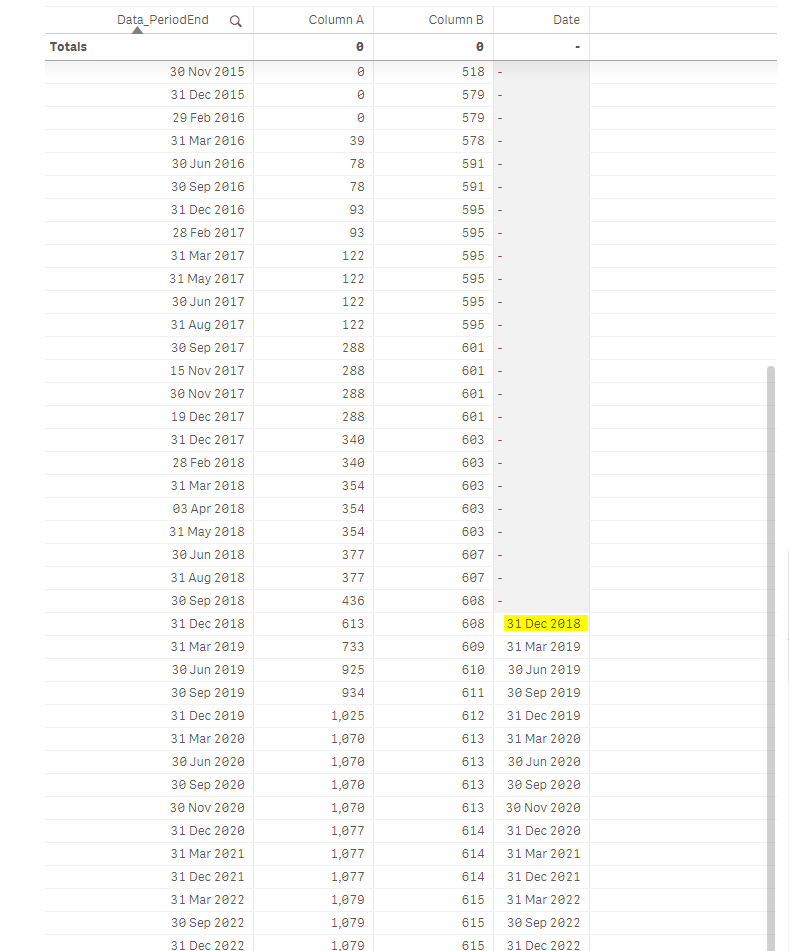# New to Qlik Sense

Discussion board where members can get started with Qlik Sense.

Announcements
See why BI users voted Qlik #1 in 11 categories. GET REPORT
cancel
Showing results for
Did you mean:
HighlightedContributor III

## Return a specific Break Even Date from Table

Hi guys,

I am trying to calculate break even in a chart and I want to return just the first value when my Column A > Column B.

if(if(Rangesum(above(\$(vRDERDist),0,rowno()))>Rangesum(above(\$(vRDERCost),0,rowno())),1,0)=1,Date(min(Data_PeriodEnd)),null())

This is the expression I have in the chart at the moment that gives me every date if Column A > Column B.

How do I change the formula if I want to return the first data where Column A > Column B ?Labels (5)

• ### rangesum

10 Replies
HighlightedMVP

May be something like this

```If(

If(RangeSum(Above(\$(vRDERDist), 0, RowNo())) > RangeSum(Above(\$(vRDERCost), 0, RowNo())), 1, 0) = 1

and

Above(If(RangeSum(Above(\$(vRDERDist), 0, RowNo())) > RangeSum(Above(\$(vRDERCost), 0, RowNo())), 1, 0)) = 0

, Date(Min(Data_PeriodEnd)), Null())```

This might not be the best way to do it, but without seeing a app to test with, this is the best I can think of.

HighlightedContributor III

It works in the chart but what if I want to show that single Date in a chart like the one below with the companies.

Do I have to use aggregation around every rangesum ?

 Asset Name Date Break Even Company 1 Company 2 Company 3 Company 4

```If(

If(RangeSum(Above(\$(vRDERDist), 0, RowNo())) > RangeSum(Above(\$(vRDERCost), 0, RowNo())), 1, 0) = 1

and

Above(If(RangeSum(Above(\$(vRDERDist), 0, RowNo())) > RangeSum(Above(\$(vRDERCost), 0, RowNo())), 1, 0)) = 0

, Date(Min(Data_PeriodEnd)), Null())```

Thanks

Michele

HighlightedMVP

@mikecherry wrote:

It works in the chart but what if I want to show that single Date in a chart like the one below with the companies.

It works in the chart, but it doesn't work in the chart? What do you mean? Why does it work and why does it not work?

HighlightedContributor III

If I copy the same expression into a chart showing Companies (like in my prior post) rather than dates it won't work anymore as I guess I have to use aggregation formulas around my expressions.

HighlightedMVP

Try this

```Aggr(
If(

If(RangeSum(Above(\$(vRDERDist), 0, RowNo())) > RangeSum(Above(\$(vRDERCost), 0, RowNo())), 1, 0) = 1

and

Above(If(RangeSum(Above(\$(vRDERDist), 0, RowNo())) > RangeSum(Above(\$(vRDERCost), 0, RowNo())), 1, 0)) = 0

, Date(Min(Data_PeriodEnd)), Null())

, Company, Data_PeriodEnd)```
HighlightedContributor III

Looks like is working. I'll do a little bit of testing and let you know.

Thanks a lot

HighlightedMVP

Sounds good 🙂

HighlightedContributor

Quick question on the previous solution re: breakeven date calculation.

How can I apply a similar approach if my variable is a calculated?

Ex: If need formulas to derive the equivalents of "vRDERDist" and "vRDERCost", do I add the smaller formula into the longer string that you shared?

HighlightedContributor

I created variables and successfully inserted them into the break even formula.

Unfortunately, the formula is returning the dates when values "broke-even" and became negative, as opposed to the dates that they "broke even" in the process of turning from negative to positive.

How can I address that in the formula?